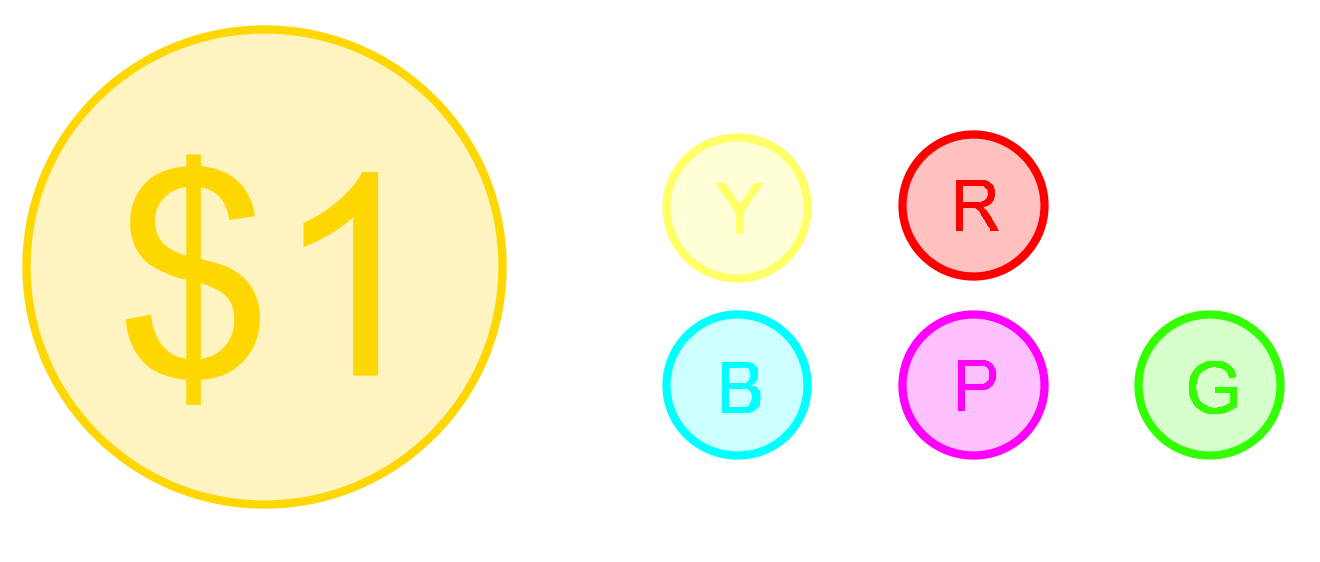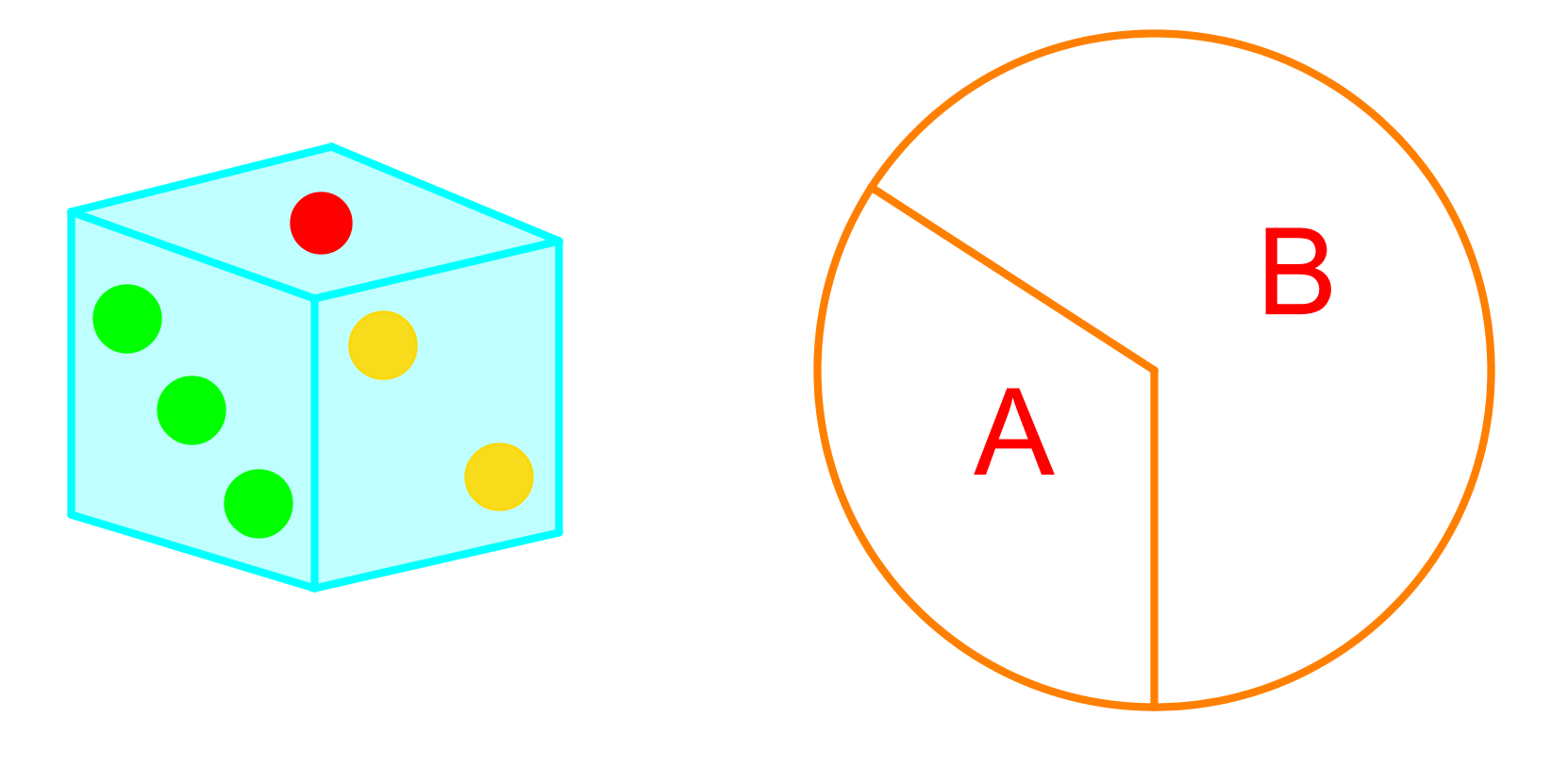# Organizing outcomes

### Organizing outcomes

In this section, we explain how to identify independent and random events. Two events are independent when the outcome of one event does not impact the outcome of the other event. An event is random when each outcome has an equal chance of occurring. In this section we practice determining the outcomes of two independent events and organizing these outcomes using tables and tree diagrams.

#### Lessons

• Introduction
a)
How to draw a tree diagram?

• 1.
Maggie flips a coin and then chooses one of five gobstoppers: yellow (Y), red (R), blue (B), purple (P), and green (G)a)
Draw a tree diagram to organize the outcomes of these two events.

b)
What is the sample space for this experiment?

c)
Are the outcomes independent? Explain why.

• 2.
Teresa rolls a 6-sided dice and then spins the spinner.a)
Organize the outcomes of these two events in a table.

b)
How many possible outcomes are there?

c)

• 3.
John, May and Betty are in a race.
a)
Show all possible outcomes in an organized list.

b)
How many possible outcomes are there if John finishes second in the race?

• 4.
Ian did a survey on how many computers a family owns in his neighbourhood. Below is the data he collected:
4, 2, 1, 5, 3, 3, 2, 4, 4, 2, 3, 1
a)
Organize the data in a frequency table.

b)
Organize the date in a dot plot.